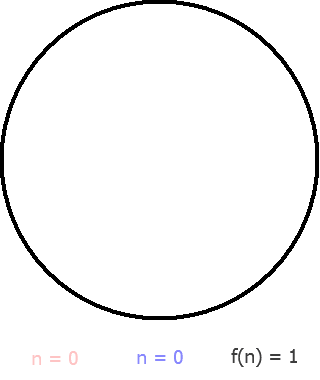# Lazy caterer’s sequence

This is one of the problems asked by a guest speaker from CMI.The lazy caterer’s sequence, formally known as the central polygonal numbers, describes the maximum number of pieces of a circle can be cut into by straight lines. For example, three cuts across a pancake will produce six pieces if the all the cuts meet at the center, but seven if they do not. This problem can be formalized mathematically as one of counting the cells in an arrangement of lines.

Now this problem was featured in codefight as the problem of the day which is how i came to know about the sequence. The question was

A cake is sliced with n straight lines. Calculate the maximum number of pieces the cake can have.
Codefight

So what is says is that-Lazy_Caterer’s_Sequence_(Cuts)

1 cut = 2 Pieces
2 cut = 4 Pieces
3 cut = 7 Pieces and so on

Solution:

So the series that finally comes up is
2,4,7,11,16,23….. and the corresponding cuts being
1,2,3, 4, 5, 6

Taking n=3 as example
we see that the series sum coming is 3 +(3-1 ) + (3-2) + (3-3) = 6 (WRONG)
Do note that no matter how many cuts we make there is always an additional piece which we take as the cake itself. We will have one piece even if we do not make any cuts. Adding that additional 1 will get out solution right.

Hence taking 3 as n we get – 1+ 3 + (3-1) + (3-2) + (3-3) =7
taking 5 as n we get – 1+ 5 + 4 + 3 +2 + 1 +0 = 16

Taking this in mind we can code the following (Java)

`public class Cake { `

`public static int calculate(int n) { `

`int sum=1; `

`for ( int i=n ; i >0 ; i--) {`

` sum=sum+i; `

`} `

`return sum; `

`} `

`}`

Now we can simplify the solution by applying the formula for Arithmetic Progression and make it

1+ n*(n+1)/2
we can convert it into the following code (Python)

`def CakeSlice(n): `

`sum=int(1+(n*(n+1)/2)) `

`return sum`

### Sliding Sidebar